# Risk Management-Section 2: Risk-Reward Ratio

Section 2: Risk-Reward Ratio

Risk-Reward ratio refers to how much money/pips you are risking in comparison to what you are willing to make on each trade.

For instance, if you are risking 30 pips on any given trade and you are willing to make 60 pips on that same trade, the risk-reward ratio here would be 2:1, meaning you are only willing to take a trade where the reward ratio is twice as large as the amount risked. A 1:2 RR ratio means that the trader is willing to make half the amount he is risking, this would be a 40 pips stop loss and a take profit level of 20 pips.

Obviously the higher the RR ratio for a set of trades, the better the results we will have with such system.

It is difficult to judge any system just by this characteristic because there are other important variables such as system accuracy*. However, system accuracy is something we do not have control of, you might be able to know the accuracy for a set of historical trades, but it doesn't necessary mean you will get the same accuracy for the next 100 trades.

*System accuracy refers to the percentage of winning trades out of an n-sample of trades.

On the other hand, we do have control over the RR ratio used on any given trade.

Like the saying from Warren Buffet “Risk comes from not knowing what you are doing” I would rather think of it this way “Risk comes from taking uncontrolled chances”. Do not try to control something that is uncontrollable (system accuracy).

To illustrate what we are telling you, let us analyze the outcome generated by a system with different RR ratios and different system accuracy. All systems are analyzed over the same sample of trades with the same sequence of winning and losing trades. It is hard to visualize the effect of this without specific examples so take some time to go through the ones below.

We will start with a system that is right 70% of the time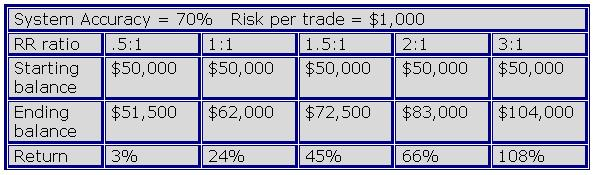[Table 1]

With such good system accuracy, a system that uses a RR ratio 0.5:1 barely makes money, returning only 3%. Just a few systems make it to this accuracy. If using a RR ratio 0.5:1 a trader would need an accuracy of over 80% to make decent profits. In contrast, other RR ratios produce a lot better results.

NOTE: Most of the time there is a trade off between the risk reward ratio and the system accuracy. It would be almost impossible to achieve a system accuracy of 70% with a 3:1 RR ratio (it is possible to achieve such system accuracy with RR ratios of 2:1, 1.5:1 or 1:1).

A system that is right 60% of the time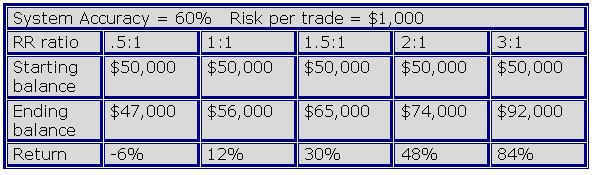[Table 2]

With an accuracy of 60%, which is exceptional, the system that uses a RR ratio of .5:1 has a negative return. This is something we cannot afford, with such an effective system we need to make money.

Higher RR ratios make money.

The system we trade has an accuracy of around 55-65%, and we always use 2:1 or higher RR ratios.

A system that is right 50% of the time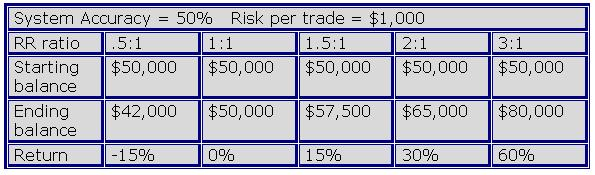[Table 3]

A system accuracy of 50% is excellent if we apply the right RR ratio. With such accuracy a system that uses a RR ratio of 1:1 (a trader risks the same amount of money he is willing to make) barely makes it to break even, this is obvious since the trader is risking the same amount of money he is willing to make and the system is right 50% of the time. Higher risk and reward ratios make money.

A system that is right 40% of the time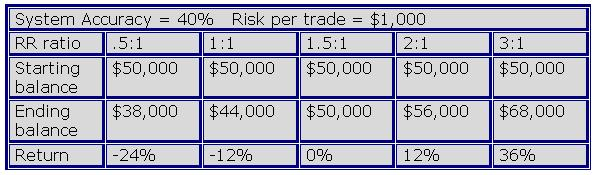[Table 4]

Most system accuracies are between 50 and 40%. At this accuracy, a system with a RR ratio of 1.5:1 breaks even. Notice also, how a system with a RR of 3:1 still makes a great return of 36%.

A system that is right 30% of the time[Table 5]

The losses incurred by the lower RR ratios are unbearable with this system accuracy. Note also the system that uses a RR ratio of 2:1 ends up with a negative return. In fact, a system with a RR ratio of 2:1 needs only a system accuracy of 36% to break even. A RR ratio of 3:1 still makes money.

A system that is right 25% of the time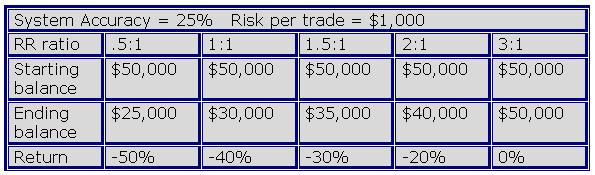[Table 6]

At this system accuracy, the system with the highest RR ratio breaks even. This means that we only need 5 trades out of 20 to make money using a system with a RR ratio of 3:1.

Are you still with us? OK - All these results lead us to a number of important conclusions:

1. As mentioned before, the accuracy of most systems are around 50%, if we use a RR ratio of 2:1, the system could make around a 30% return over a sample of 30 trades, not bad is it? In addition as we saw in an earlier lesson, if money management is applied the return could be greater.

2. We talked about the characteristics of a trading system, which are: the RR ratio and the accuracy. We know that the future accuracy is unknown, we might know the accuracy for any given set of historical trades, but will never know the accuracy for the next set of trades, thus it cannot be manipulated. What is known and can be manipulated is the RR ratio of our system. By adjusting (up) the RR ratio of our system the odds of profitability increase substantially.

However, we need to have something in mind, in most cases there is a negative correlation (or trade off) between the RR ratio used and the accuracy of any system.

Most of the time, the higher the RR ratio, the lower the accuracy and the lower the RR ratio, the higher the accuracy. You can see this when testing a trading system, the closer the take profit order is to the entry price the better accuracy you will get.

3. Most of us grew up with the idea the anything below 70% of effectiveness on any task is a failure (i.e. our grades at primary school). However, it is way different in real life, take for instance professional baseball players, they get very well paid if they hit the ball a little above 30% of the time. In addition, the same is applied for traders, with a system that is right 50% of the time, we all could get great results in our trading careers if we use a good RR ratio.

I hope I have convinced you that a good accuracy is not always the right path. We are not saying however that the systems that have a good accuracy and low RR ratio are always losing systems. There are systems that have good accuracy (with a lower RR ratio) that produce outstanding results. We attempt to find the right combination of the two that maximize the profit potential.